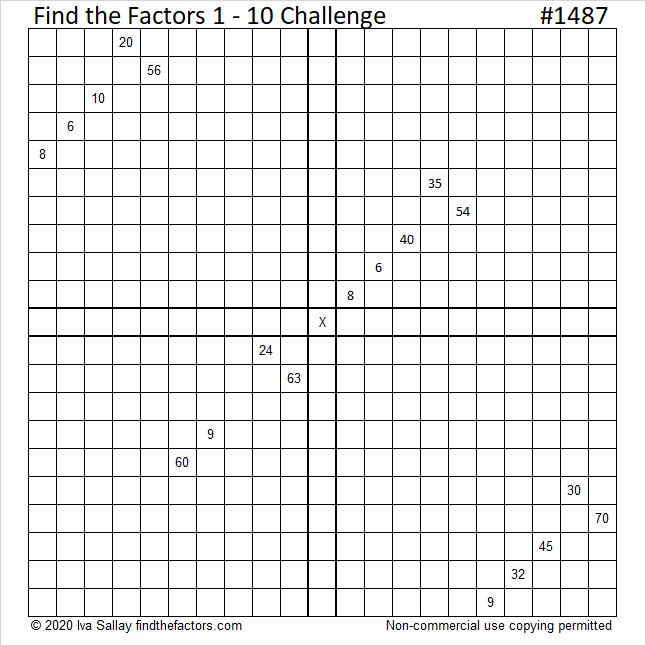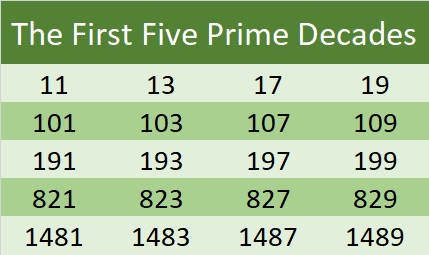# 1487 A Challenging Puzzle

### Today’s Puzzle:

This puzzle is like four out-of-order 1 – 10 multiplication tables that work together. The clues in the puzzle need to be the products of the numbers you write. It won’t be easy, but use logic to solve it. Good luck!Print the puzzles or type the solution in this excel file: 10 Factors 1478-1487

### Factors of 1487:

• 1487 is a prime number.
• Prime factorization: 1487 is prime.
• 1487 has no exponents greater than 1 in its prime factorization, so √1487 cannot be simplified.
• The exponent in the prime factorization is 1. Adding one to that exponent we get (1 + 1) = 2. Therefore 1487 has exactly 2 factors.
• The factors of 1487 are outlined with their factor pair partners in the graphic below.How do we know that 1487 is a prime number? If 1487 were not a prime number, then it would be divisible by at least one prime number less than or equal to √1487. Since 1487 cannot be divided evenly by 2, 3, 5, 7, 11, 13, 17, 19, 23, 29, 31, or 37, we know that 1487 is a prime number.

### Other Facts about the Number 1487:

1487 is the difference of two squares:
744²-743² = 1487.

1487 is the third prime number in the fifth prime decade. See it in the list below: